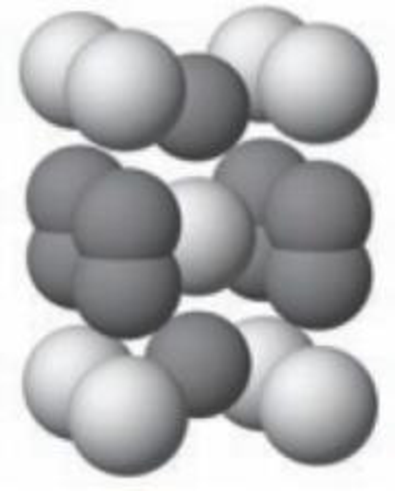Chapter 12, Problem 44GQ

Chapter
Section
Textbook Problem

The unit cell shown here is for calcium carbide. How many calcium atoms and how many carbon atoms are in each unit cell? What is the formula of calcium carbide? (Calcium ions are silver in color and carbon atoms are gray.)Unit cell for calcium carbide

Interpretation Introduction

Interpretation:

The number of calcium atoms, the number of carbon atom in unit cell and formula of calcium carbide has to be calculated.

Concept introduction:

Body Centered Cubic Structure:

In simple cubic crystal structure, eight atoms is placed in the corner of the cube and one atom in the center of the cube, therefore it is called as body-centered cubic.

Explanation

The unit cell for calcium is body- centered cubic. There are atoms at each of the corners of a cube and one atom embedded in the middle of the cube.

Number of Calcium atoms per unit is given below,

It has 8 atoms in the corner, 1 atom in the body.

Number of atoms per unit=(8corneratoms×1/8+1bodyatoms×1)Number of atoms per unit=2

There are ten carbon atoms per unit cell

Still sussing out bartleby?

Check out a sample textbook solution.

See a sample solution

The Solution to Your Study Problems

Bartleby provides explanations to thousands of textbook problems written by our experts, many with advanced degrees!

Get Started

Children who don't drink milk often have lower bone density than than those who do? T F

Nutrition: Concepts and Controversies - Standalone book (MindTap Course List)

What is a chemical reaction?

Biology: The Dynamic Science (MindTap Course List)

How can you explain the Algol paradox?

Horizons: Exploring the Universe (MindTap Course List)